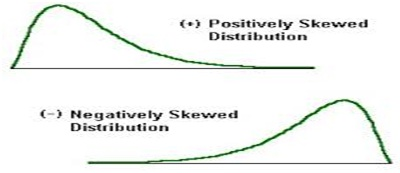# Positive Skewness

Posted in Statistics, Total Reads: 13708

## Definition: Positive Skewness

Skewness is a measure of the extent to which the probability distribution of a real-valued random variable leans on any side of the mean of the variable. A probability distribution does not need to be a perfect bell shaped curve.

The right and the left side may not be mirror images. Skewness measures this extent of asymmetry. If the data is positively skewed then it will have a much longer right tail than the left tail. In this case, mean and median will be greater than mode. In most of the cases, mean will be greater than median.

Hence,

Mean ≥ Median > Mode

Positively skewed data is also called right skewed, right-tailed, skewed to the right.

Similarly, if the data is skewed to the left then it will have a much longer left tail and the data is called negatively skewed, left-skewed, left-tailed or simply tailed to the left. Also in this case,

Mean ≤ Median < Mode

One popular measure of skewness is Pearson’s First Coefficient, which is the ratio of the difference between mean and mode to the standard deviation. If the data is positively skewed, the coefficient is positive; else it is negative for negatively skewed data. An example of positively skewed data is the life of bulbs. The smallest value can be zero, and the long life of the bulbs will make the distribution skewed towards the right.Hence, this concludes the definition of Positive Skewness along with its overview.

Browse the definition and meaning of more terms similar to Positive Skewness. The Management Dictionary covers over 7000 business concepts from 6 categories.

Search & Explore : Management Dictionary

Similar Definitions from same Category: